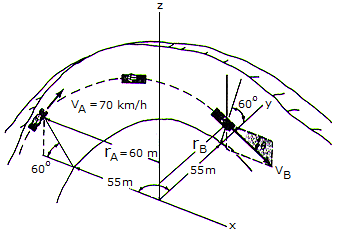# Engineering Mechanics - KOP: Impulse and Momentum - Discussion

### Discussion :: KOP: Impulse and Momentum - General Questions (Q.No.8)

8.A toboggan and rider, having a total mass of 150 kg, enter horizontally tangent to a 90° circular curve with a velocity VA and the angleof "descent," measured from the horizontal in a vertical xz plane, at which the toboggan exits at B. Neglect friction in the calculation. The radius rB equals 57 m.

 [A]. vB = 21.9 m/s,= 20.9° [B]. vB = 23.4 m/s,= 29.0° [C]. vB = 20.7 m/s,= 8.84° [D]. vB = 20.3 m/s,= 7.37°

Explanation:

No answer description available for this question.